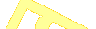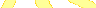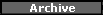SubscribeThe MP2K Update!MagazineFeaturesMapPoint 2013 Press Releases MapPoint Forums Companies Link to MP2Kmag Wish List MapPoint Trial AuthorsEarlier ContentPast News Items Past What's New AnnouncementsOrderMapPoint 2013
Programming MapPoint in .NET
MapPoint BookSpatial CommunityARTICLES#### Determing Whether A Point Is Located Inside Polygon

Wilfried Mestdagh wrote this article on determing whether a vehicle is located inside or outside a convex polygon. "Simplify the polygon by make triangles from it one by one, until we have a triangle where the point is inside. At the end we only have to verify if the point is in the last triangle."

 This article explains a method to calculate if a position is in or outside a convex polygon. It does not make use of floating point numbers to optimize for speed as much as possible. To transform Latitude / Longitude pairs into X/Y coordiantes, just multiply them with a given factor, for example 100000. 1/100000 of a degree gives precision of around 1 meter which is already better than any GPS receiver. If you just want to download the complete project, included with demo executable and source code then click here. To make the calculations easy we will reduce the polygon to triangles by replacing each time two successive sides by a new one, provided that the triangle formed by the two polygon sides and the new side does not contain the point.Simplify the polygon by make triangles from it one by one, until we have a triangle where the point is inside.
 At the end we only have to verify if the point is in the last triangle.The last triangle.
 So we reduce the whole problem to a simple problem if a certain triangle a certain point has in itself. Now, to move the triangle and also the point in such a way that the point is on the origin of the coordinate axis (0,0) we again simplify the calculations.Moving the polygon to the origin 0,0 of coordinates.
 We search the sides intercepting the Y axis. If we don't find them then the zero point is certainly not in the triangle. The 2 intercept points have to each be on another side of the X axis or on the X axis itself. The calculation of a straight line going through the points X1, Y1 and X2, Y2 is: `z (x) = (y2 - y1) / (x2 - x1) x - (y2 - y1) / (x2 - x1) + y1` Now we have to see if the side is intercepting the Y axis. If x1 = x2 then this cannot be, so we can drop the case. To find the intercept point of a straight line with the Y axis we find to compute z(0): `z(0) = (y1 - y2) / (x2 - x1) + y1` This intercept point is on the side and not on the straight line past the side if sng(x1 <> sng(x2). Finally we have to find out if the side is intercepting the Y axis above or below the X axis. We call the intercept point z(0) (see above). So we have to find the sign of x(0). So z(0) >= 0. `(y1 - y2) / (x2 - x1) * x1 + y1 >= 0` If x2 < x2, then x2 - x1 > 0 and then: `(y1 - y2) * x1 >= y1( - y2) [E1]` If x1 > x2, then x2 - x1 < 0, and then: `(y1 - y2) * x1 <= y1(x1 - x2) [E2]` So the intercept point is above the X axis if E1 or E2 is true. Below is the complete code written in Delphi. You can translate it into the programming language of your choice and if you do so please post in the discussion thread for this article. unit uPolygon; interface uses Windows; type TCoord = record X: integer; Y: integer; end; type TAngles = record NoOfPoints: integer; Points: array [0..50] of TCoord end; type TPolygon = class private Angles: TAngles; SearchPoint: TCoord; Polygon: array [0..50] of TCoord; procedure ReadCoordinates; procedure RemoveAngle(AngleNo: integer); function UpperXAxis(P1, P2: TCoord): boolean; function YInterceptPoint(P1, P2: TCoord): boolean; function PointInTriangle(AnglePoint1, AnglePoint2, AnglePoint3: TCoord): boolean; function TransformPolygon: boolean; public CouldNotResolve: boolean; Points: array[0..50] of TPoint; AngleCount: integer; VehiclePosition: TPoint; function IsDrivingOutsidePolygon: boolean; end; implementation procedure TPolygon.ReadCoordinates; var i: integer; begin for i := 0 to AngleCount - 1 do begin Polygon[i].X := Angles.Points[i].X; Polygon[i].Y := Angles.Points[i].Y; end; end; procedure TPolygon.RemoveAngle(AngleNo: integer); var Angle: integer; begin for Angle := AngleNo + 1 to AngleCount - 1 do Polygon[Angle - 1] := Polygon[Angle]; Dec(AngleCount); end; function TPolygon.UpperXAxis(P1, P2: TCoord): boolean; begin if P1.X < P2.X then Result := (P1.Y - P2.Y) * P1.X >= P1.Y * (P1.X - P2.X) else Result := (P1.Y - P2.Y) * P1.X <= P1.Y * (P1.X - P2.X); end; function TPolygon.YInterceptPoint(P1, P2: TCoord): boolean; begin if P1.X = P2.X then Result := False else if P1.X < 0 then Result := P2.X >= 0 else Result := P2.X <= 0; end; function TPolygon.PointInTriangle(AnglePoint1, AnglePoint2, AnglePoint3: TCoord): boolean; var P1, P2, P3: TCoord; Intercept1, Intercept2, Intercept3, A, B, ItIsIn: boolean; begin P1.X := AnglePoint1.X - SearchPoint.X; P2.X := AnglePoint2.X - SearchPoint.X; P3.X := AnglePoint3.X - SearchPoint.X; P1.Y := AnglePoint1.Y - SearchPoint.Y; P2.Y := AnglePoint2.Y - SearchPoint.Y; P3.Y := AnglePoint3.Y - SearchPoint.Y; Intercept1 := YInterceptPoint(P1, P2); Intercept2 := YInterceptPoint(P2, P3); Intercept3 := YInterceptPoint(P3, P1); ItIsIn := False; if Intercept1 and Intercept2 then begin A := UpperXAxis(P1, P2); B := UpperXAxis(P2, P3); if (A xor B) then ItIsIn := True; end; if Intercept1 and Intercept3 and not ItIsIn then begin A := UpperXAxis(P1, P2); B := UpperXAxis(P3, P1); if (A xor B) then ItIsIn := True; end; if Intercept2 and Intercept3 and not ItIsIn then begin A := UpperXAxis(P2, P3); B := UpperXAxis(P3, P1); if (A xor B) then ItIsIn := True; end; Result := ItIsIn; end; function TPolygon.TransformPolygon: boolean; var AngleGone: boolean; Angle1, Angle2, Angle3: integer; begin if AngleCount > 3 then begin Angle1 := 0; while (Angle1 < AngleCount) and (AngleCount > 3) do begin AngleGone := true; while AngleGone and (AngleCount > 3) do begin if Angle1 = AngleCount - 1 then begin Angle2 := 0; Angle3 := 1; end else if Angle1 + 1 = AngleCount - 1 then begin Angle2 := AngleCount - 1; Angle3 := 0; end else begin Angle2 := Angle1 + 1; Angle3 := Angle1 + 2; end; AngleGone := not PointInTriangle(Polygon[Angle1], Polygon[Angle2], Polygon[Angle3]); if AngleGone then RemoveAngle(Angle2); end; Angle1 := Angle1 + 1; end; end; Result := AngleCount = 3; end; function TPolygon.IsDrivingOutsidePolygon: boolean; var n: integer; begin CouldNotResolve := False; SearchPoint.Y := VehiclePosition.Y; SearchPoint.X := VehiclePosition.X; for n := 0 to AngleCount - 1 do begin Angles.Points[n].X := Points[n].X; Angles.Points[n].Y := Points[n].Y; end; ReadCoordinates; Angles.NoOfPoints := AngleCount; if (TransformPolygon) then Result := not PointInTriangle(Polygon, Polygon, Polygon) else begin Result := False; // transformation not possible CouldNotResolve := True; end; end; end.

Discuss this story in the forum.Author: Wilfried Mestdagh
Email: wilfried(AT)mestdagh.biz
Wilfried Mestdagh works as software engineer at the company Sonal in Mortsel, Belgium. His main work is writing software for fleet management and onboard computers. Fleet management is mainly written in Delphi and C# while the onboard computers are mostly programmed in C. His department started years ago for specializing in tracking and tracing security and dangerous transport vehicles, but it is grown to satisfy a very wide of vehicle / truck fleet customers.MP2Kmag InternetRecent DiscussionResourcesBrowse GIS books and periodicalsFind a MapPoint Partner or ConsultantReal Estate Columbia MO Homes for Sale Want Your Site To Appear Here?